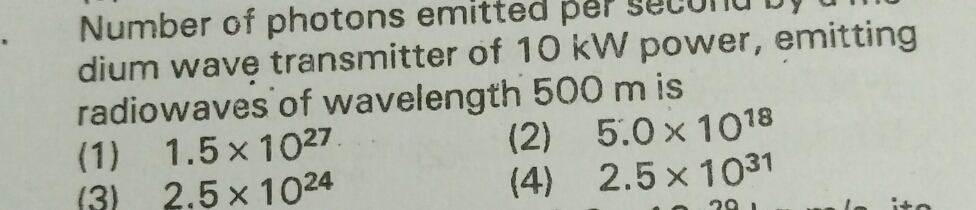# No of photon emitted per second by a medium wave transmitter of 10 kW power, emitting radiowaves of wavelength 500 m5 years ago
Dear Anjali

Power of the medium wave transmitter, P= 10 kW = 104 W = 104J/s

Hence, energy emitted by the transmitter per second, E= 104

Wavelength of the radio wave, = 500 m

The energy of the wave is given as:

h* c/Lambda

Where,

h= Planck’s constant = 6.6* 10^-34 J sec

c= Speed of light = 3 × 108m/s

E1 = 6.6*10 ^-34 * 3* 10^8/500 = 3.96* 10^-28

Let n be the number of photons emitted by the transmitter.

nE1= E

n = E/E1

= 10^4/3.96 * 10^-28 = 2.525* 10^31

Regards